International
Tables for
Crystallography
Volume C
Mathematical, physical and chemical tables
Edited by E. Prince

International Tables for Crystallography (2006). Vol. C, ch. 7.5, pp. 667-668

## Section 7.5.7. Optimization of counting times

A. J. C. Wilsona

aSt John's College, Cambridge CB2 1TP, England

### 7.5.7. Optimization of counting times

| top | pdf |

There have been many papers on optimizing counting times for achieving different purposes, and all optimization procedures require some knowledge of the distributions of counts or counting rates; often only the mean and variance of the distribution are required. It is also necessary to know the functional relationship between the quantity of interest and the counts (counting rates, intensities) entering into its measurement. Typically, the object is to minimize the variance of some function of the measured intensities, say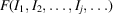. General statistical theory gives the usual approximation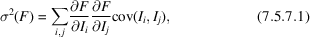where cov(Ii, Ij) is the covariance of Ii and Ij if, and is the variance of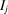,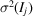, if i = j. There is very little correlation2 between successive intensity measurements in diffractometry, so that cov(Ii, Ij) is negligible for. Equation (7.5.7.1)becomes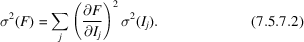These equations are strictly accurate only if F is a linear function of the I's, a condition satisfied for the integrated intensity, but for few other quantities of interest. In most applications in diffractometry, however, the contribution of each Ij is sufficiently small in comparison with the total to make the application of equations (7.5.7.1)and (7.5.7.2)plausible. Any proportionality factors(Section 7.5.1) can be absorbed into the functional relationship between F and the Ij's.

The object is to minimize σ2(F) by varying the time spent on each observation, subject to a fixed total time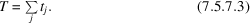It is simplest to regard the total intensity and the background intensity as separate observations, so that in (7.5.7.2)the sum is over n background' and n total' observations. Withexpressed as a counting rate, its variance is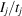[equation (7.5.3.8)], so that (7.5.7.2)becomes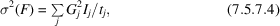where for brevity G has been written for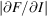. The variance of F will be a minimum if, for any small variations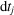of the counting times,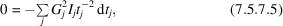subject to the constancy of the total time T. There is thus the constraint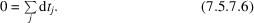These equations are consistent if for all j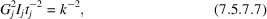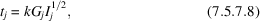where k is a constant determined by the total time T: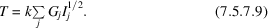The minimum variance is thus achieved if each observation is given a time proportional to the square root of its intensity. A little manipulation now gives for the desired minimum variance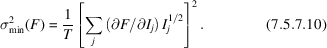The minimum variance is found to be a perfect square, and the standard uncertainty takes a simple form.

Here, the optimization has been treated as a modification of fixed-time counting. However, the same final expression is obtained if the optimization is treated as a modification of fixed-count timing (Wilson, Thomsen & Yap, 1965).

Space does not permit detailed discussion of the numerous papers on various aspects of optimization. If the time required to move the diffractometer from one observation position to another is appreciable, the optimization problem is affected (Shoemaker & Hamilton, 1972, and references cited therein). There is some dependence on the radiation (X-ray versus neutron) (Shoemaker, 1968; Werner, 1972a,b). A few other papers of historical or other interest are included in the list of references, without detailed mention in the text: Grant (1973); Killean (1972, 1973); Mack & Spielberg (1958); Mackenzie & Williams (1973); Szabó (1978); Thomsen & Yap (1968); Zevin, Umanskii, Kheiker & Panchenko (1961).

### References

Grant, D. F. (1973). Single-crystal diffractometer data: the on-line control of the precision of intensity measurement. Acta Cryst. A29, 217.
Killean, R. C. G. (1972). The a priori optimization of diffractometer data to achieve the minimum average variance in the electron density. Acta Cryst. A28, 657–658.
Killean, R. C. G. (1973). Optimization of scan procedure for single-crystal X-ray diffraction intensities. Acta Cryst. A29, 216–217.
Mack, M. & Spielberg, N. (1958). Statistical factors in X-ray intensity measurements. Spectrochim. Acta, 12, 169–178.
Mackenzie, J. K. & Williams, E. J. (1973). The optimum distribution of counting times for determining integrated intensities with a diffractometer. Acta Cryst. A29, 201–204.
Shoemaker, D. P. (1968). Optimization of counting times in computer-controlled X-ray and neutron single-crystal diffractometry. Acta Cryst. A24, 136–142.
Shoemaker, D. P. & Hamilton, W. C. (1972). Further remarks concerning optimization of counting times in single-crystal diffractometry: rebuttal to Killean; consideration of background counting and slewing times. Acta Cryst. A28, 408–411.
Szabó, P. (1978). Optimization of the measuring time in diffraction intensity measurements. Acta Cryst. A34, 551–553.
Thomsen, J. S. & Yap, F. Y. (1968). Simplified method of computing centroids of X-ray profiles. Acta Cryst. A24, 702–703.
Werner, S. A. (1972a). Choice of scans in X-ray diffraction. Acta Cryst. A28, 143–151.
Werner, S. A. (1972b). Choice of scans in neutron diffraction. Acta Cryst. A28, 665–669.
Wilson, A. J. C., Thomsen, J. S. & Yap, F. Y. (1965). Minimization of the variance of parameters derived from X-ray powder diffractometer line profiles. Appl. Phys. Lett. 7, 163–165.
Zevin, L. S., Umanskii, M. M., Kheiker, D. M. & Panchenko, Yu. M. (1961). K voprosu o difraktometricheskikh priemah pretsizionnyh izmerenii elementarnyh yacheek. Kristallografiya, 6, 348–356.